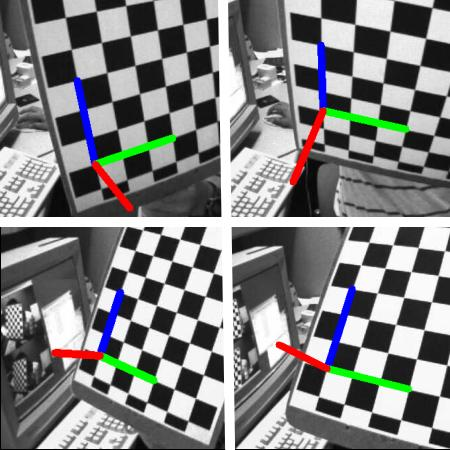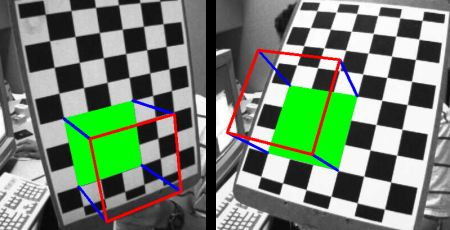# OpenCV-Python姿势估计

## 姿势估计基础

``````import numpy as np
import cv2 as cv
import glob

# 读取事先存好的数据
with np.load('B.npz') as X:
mtx, dist, _, _ = [X[i] for i in ('mtx','dist','rvecs','tvecs')]
``````

``````def draw(img, corners, imgpts):
corner = tuple(corners.ravel())
img = cv.line(img, corner, tuple(imgpts.ravel()), (255,0,0), 5)
img = cv.line(img, corner, tuple(imgpts.ravel()), (0,255,0), 5)
img = cv.line(img, corner, tuple(imgpts.ravel()), (0,0,255), 5)
return img
``````

``````criteria = (cv.TERM_CRITERIA_EPS + cv.TERM_CRITERIA_MAX_ITER, 30, 0.001)
objp = np.zeros((6*7,3), np.float32)
objp[:,:2] = np.mgrid[0:7,0:6].T.reshape(-1,2)

axis = np.float32([[3,0,0], [0,3,0], [0,0,-3]]).reshape(-1,3)
``````

``````for fname in glob.glob('left*.jpg'):
gray = cv.cvtColor(img,cv.COLOR_BGR2GRAY)
ret, corners = cv.findChessboardCorners(gray, (7,6),None)

if ret == True:
corners2 = cv.cornerSubPix(gray,corners,(11,11),(-1,-1),criteria)

# 找到旋转和平移向量
ret,rvecs, tvecs = cv.solvePnP(objp, corners2, mtx, dist)

# 投射 3D 点到平面图像上
imgpts, jac = cv.projectPoints(axis, rvecs, tvecs, mtx, dist)

img = draw(img,corners2,imgpts)
cv.imshow('img',img)
k = cv.waitKey(0) & 0xFF
if k == ord('s'):
cv.imwrite(fname[:6]+'.png', img)

cv.destroyAllWindows()
``````### 渲染立方体

``````def draw(img, corners, imgpts):
imgpts = np.int32(imgpts).reshape(-1,2)

# 将底面绘制为绿色
img = cv.drawContours(img, [imgpts[:4]],-1,(0,255,0),-3)

# 将支柱绘制为蓝色
for i,j in zip(range(4),range(4,8)):
img = cv.line(img, tuple(imgpts[i]), tuple(imgpts[j]),(255),3)

# 将顶面绘制为红色
img = cv.drawContours(img, [imgpts[4:]],-1,(0,0,255),3)

return img
``````

``````axis = np.float32([ [0,0,0], [0,3,0], [3,3,0], [3,0,0],
[0,0,-3],[0,3,-3],[3,3,-3],[3,0,-3] ])
``````• 微信订阅
• 回顶